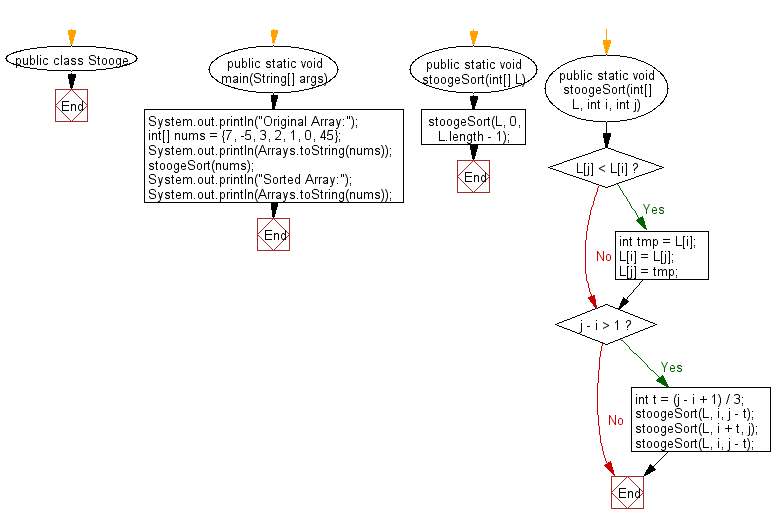﻿ Java exercises: Stooge Sort Algorithm - w3resource# Java Exercises: Stooge Sort Algorithm

## Java Sorting Algorithm: Exercise-18 with Solution

Write a Java program to sort an array of given non-negative integers using Stooge Sort Algorithm.

Stooge sort is a recursive sorting algorithm with a time complexity of O(nlog 3 / log 1.5 ) = O(n2.7095...). The running time of the algorithm is thus slower compared to efficient sorting algorithms, such as Merge sort, and is even slower than Bubble sort.

Sample Solution:

Java Code:

``````import java.util.Arrays;
public class Stooge {
public static void main(String[] args) {
System.out.println("Original Array:");
int[] nums = {7, -5, 3, 2, 1, 0, 45};
System.out.println(Arrays.toString(nums));
stoogeSort(nums);
System.out.println("Sorted Array:");
System.out.println(Arrays.toString(nums));
}

public static void stoogeSort(int[] L) {
stoogeSort(L, 0, L.length - 1);
}

public static void stoogeSort(int[] L, int i, int j) {
if (L[j] < L[i]) {
int tmp = L[i];
L[i] = L[j];
L[j] = tmp;
}
if (j - i > 1) {
int t = (j - i + 1) / 3;
stoogeSort(L, i, j - t);
stoogeSort(L, i + t, j);
stoogeSort(L, i, j - t);
}
}
}

```
```

Sample Output:

```Original Array:
[7, -5, 3, 2, 1, 0, 45]
Sorted Array:
[-5, 0, 1, 2, 3, 7, 45]
```

Flowchart:Java Code Editor:

What is the difficulty level of this exercise?

﻿

## Java: Tips of the Day

Array vs ArrayLists:

The main difference between these two is that an Array is of fixed size so once you have created an Array you cannot change it but the ArrayList is not of fixed size. You can create instances of ArrayLists without specifying its size. So if you create such instances of an ArrayList without specifying its size Java will create an instance of an ArrayList of default size.

Once an ArrayList is full it re-sizes itself. In fact, an ArrayList is internally supported by an array. So when an ArrayList is resized it will slow down its performance a bit as the contents of the old Array must be copied to a new Array.

At the same time, it's compulsory to specify the size of an Array directly or indirectly while creating it. And also Arrays can store both primitives and objects while ArrayLists only can store objects.

Ref: https://bit.ly/3o8L2KH